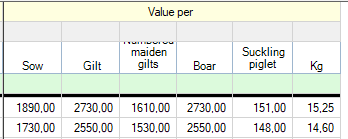Calculation of valuation change in e-control sows.

Valuation change sows

(Sows at end * Value at end) – (Sows at start * Value at start)

Valuation change pregnant days

((((Sows preg. days at end + Gilts preg. days at end)* FU per pregnant day(Note a) ) / Production factor per produced pig(Note b)) * (Value per suckling pig at end + (Price per kg suckling pig at slut * avg. weight suckling pigs at end))

-

(((((Sow preg. days at start + Gilts preg. days at start)) * FU per pregnant day(Note a) ) / Production factor per produced pig(Note b))* (Value per suckling pig at start + (Price per kg suckling pig at start * avg. weight suckling pig at start))

+

Valuation change boars

(Boars at end * Value at end) – (Boars at start * Value at start)

Valuation change maiden gilts (numbered)

( Maiden gilts at end * Value at end) – (Maiden gilts at start * Value at start)

+

Valuation change maiden gilts (location/groups)

( Maiden gilts at end * Value at end) – (Maiden gilts at start – Value at start) + ( Kg at end * Value per kg at end) – (Kg at start – Value per kg at end)

+

Valuation change suckling piglets

(((Suckling pigs at end * Production factor per suckling pig at end(Note b)) / Production factor per prod. Pig(Note b)) *( Value per suckling pig at end + (Value per kg at end * avg. weight suckling pigs at end)))

-

(((Suckling pigs at start start * Production factor per suckling pig at start(Note b)) / Production factor per prod. Pig(Note b)) * ( Value per suckling pig at start + (Value per kg at start * Avg. weight suckling pigs at start)))

+

Valuation change nursery (if they are part of the sow herd calculation)

(((Nursery pigs at end * Production factor per pig at end(Note b)) / Production factor per prod. Pig(Note b)) *( Value per pig end + (Value per kg end * Avg. weight at end)))

-

(((Nursery pigs at start * Production factor per pig at start(Note b)) / Production factor per prod. Pig(Note b)) * ( Value per pig at start + (Value per kg at start * Avg. weight at start)))

Notes

a) FE per pregnant day

Is a conversion table which is used to transform gestation days into pigs of production weight.

b) Production factor

Is a conversion table used to transform pigs of a given weight into pigs with production weight.

If stock take values isn’t entered

As a starting point, the status values that are entered directly in the status windows are used together with the number. If these values are not entered, the program will try to calculate some values based on relevant exits of animals.

Which values to be entered as stock take values?

It is recommended always to use same method for value assessment, otherwise there will occur fluctuations in the valuation change which is not related to the herd.

Where to enter the values in PigVision

Go to Sows boars – Stocktake where stock take is entered.

In the sow herd some groups of animal are not numbered as individuals. These animals are valuated at stock take with a price per life and a price per kg.

Suckling pigs, pigs in nursery and unnumbered maiden gilts is valuated this way.

Sows, gilts, numbered maiden gilts and breeding boars are valuated with an avg price per animal.Figure 1 Example of stock take of suckling pigs, nursery and maiden gilts.Figure 2 Example of stock take values for sows, gilts etc.

Related topics:

Stocktake

See the formula of a key figure

Calculation of valuation change, progeny